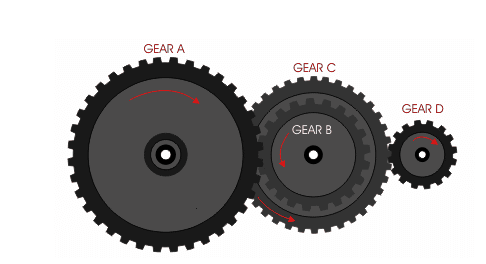News & Events

# What is the transmission ratio

In a mechanical transmission system, the ratio of the angular velocity or rotational speed of the driving wheel at the beginning and the driven wheel at the end.
Transmission ratio (i) = ratio of driving wheel speed (n1) to driven wheel speed (n2) = inverse ratio of gear index circle diameter = ratio of driven gear teeth (Z2) to driving gear teeth (Z1).
That is: i=n1/n2=D2/D1 i=n1/n2=z2/z1
For multi-stage gear transmission
1: The transmission ratio between every two shafts is calculated according to the above formula
2: The total transmission ratio from the first axis to the nth axis is calculated according to the following formula: Total transmission ratio ι=(Z2/Z1)×(Z4/Z3)×(Z6/Z5)……=(n1/n2)× (N3/n4)×(n5/n6)……For multi-stage gear transmission

Extended information

The ratio of the angular velocities of the two rotating components in the mechanism is also called the speed ratio. The transmission ratio of component a and component b is Ⅰ=ωa/ωb=na/nb, where ωa and ωb are the angular velocities (radians/sec) of components a and b, respectively; na and nb are the rotational speeds of components a and b respectively ( Rpm) (Note: a and b after ω and n are subscripts).
When the angular velocity in the formula is an instantaneous value, the obtained transmission ratio is the instantaneous transmission ratio. When the angular velocity in the formula is an average value, the obtained transmission ratio is the average transmission ratio. For most gear transmissions and friction wheel transmissions with the correct tooth profile, the instantaneous transmission ratio is unchanged; for chain transmission and non-circular gear transmission, the instantaneous transmission ratio is variable.
For meshing transmission, the transmission ratio can be expressed by the number of teeth Za and Zb of wheel a and wheel b, i=Zb/Za; for friction transmission, the transmission ratio can be expressed by the radius Ra and Rb of wheel a and wheel b, i=Rb/Ra, At this time, the transmission ratio generally means the average transmission ratio.
In hydraulic transmission, the transmission ratio of the hydraulic transmission element generally refers to the ratio of the turbine speed S and the pump wheel speed B, that is, =S/B. Hydraulic transmission elements can also be combined with mechanical transmission elements (generally with various gear trains) to obtain various transmission ratios of different values ​​(see gear trains for gear train transmission ratios).